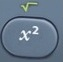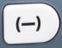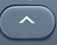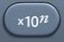# Order of Operations

Order of Operations = PEMDAS

Order of Operations
go in order of priority by the acronym PEMDAS:
1. Parentheses
2. Exponents (Powers and Roots)
3. Multiplication and Division

When operations have the same priority, the operations move from left to right.

For reasons which will become obvious in the Answer Processes below, a question involving order of operations is usually better suited to the calculator.

However, even with the calculator, you may need to use parentheses to protect the order of operations in calculations involving squaring, power, scientific notation, and other potentially confusing mathematical situations.

Question

What is the value of 3 + 8 × 4?

35

The multiplication operation has the highest priority in this mathematical expression.
8 × 4 = 32
Rewrite the expression.
3 + 8 × 4 = 3 + 32
3 + 32
The addition operation is all that remains.
3 + 32 = 35

Input Display Commentblinker clears screen
3 + 8 × 4 3+8*435 Answer
###### Order of Operations

Question

What is the value of 3 + 8 × 4 – 5²?

10

The exponent operation has the highest priority in this mathematical expression.
5² = 25
Rewrite the expression.
3 + 8 × 4 – 5² = 3 + 8 × 4 – 25
3 + 8 × 4 – 25
The multiplication operation has the highest priority in the rewritten expression.
8 × 4 = 32
Rewrite the expression.
3 + 8 × 4 – 25 = 3 + 32 – 25
3 + 32 – 25
Because the addition operation has the same priority as the subtraction operation in the rewritten expression, perform the operation on the left.
3 + 32 = 35
Rewrite the expression.
3 + 32 – 25 = 35 – 25
35 – 25

The subtraction operation is all that remains.
35 – 25 = 10

Input Display Commentblinker clears screen
3 + 8 × 4 – 53+8*4-5²10 Answer
###### Order of Operations

Question

What is the value of 3 + 8 × 4 – 25 ?

30

The root operation has the highest priority in this mathematical expression.
25 = 5
Rewrite the expression.
3 + 8 × 4 – 25 = 3 + 8 × 4 – 5
3 + 8 × 4 – 5
The multiplication operation has the highest priority in the rewritten operation.
8 × 4 = 32
Rewrite the expression.
3 + 8 × 4 – 5 = 3 + 32 – 5
3 + 32 – 5
Because the addition operation has the same priority as the subtraction operation in the rewritten expression,
perform the operation on the left.
3 + 32 = 35
Rewrite the expression.
3 + 32 – 5 = 35 – 5
35 – 5

The subtraction operation is all that remains.
35 – 5 = 30

Input Display Commentblinker clears screen
3 + 8 × 4 –25 3+8*4-√2530 Answer
###### Order of Operations

Question

What is the value of 3 + 8 × 4 – 5² + (7 – 2)?

15

The operation in parentheses has the highest priority in this mathematical expression.
(7 – 2) = 5
Rewrite the expression.
3 + 8 × 4 – 5² + (7 -2 ) = 3 + 8 × 4 – 5² + 5
3 + 8 × 4 – 5² + 5
The exponent operation has the highest priority in the rewritten expression.

5² = 25
Rewrite the expression.
3 + 8 × 4 – 5² + 5 = 3 + 8 × 4 – 25 + 5
3 + 8 × 4 – 25 + 5
The multiplication operation has the highest priority in the rewritten expression.
8 × 4 = 32
Rewrite the expression.
3 + 8 × 4 – 25 = 5 = 3 + 32 – 25 + 5
3 + 32 – 25 + 5
Because the addition, subtraction, and addition operations have the same priority in the rewritten expression, perform the operation on the left.
3 + 32 = 35
Rewrite the expression.
3 + 32 – 25 + 5 = 35 – 25 + 5
35 – 25 + 5
Because the subtraction operation has the same priority as the addition operation in the rewritten expression, perform the operation on the left.
35 – 25 = 10
Rewrite the expression.
35 – 25 + 5 = 10 + 5
10 + 5
The addition operation is all that remains.
10 + 5 = 15

Input Display Commentblinker clears screen
3 + 8 × 4 – 5+7-23+8*4-5²+(7-2)15 Answer
###### Order of Operations

Question
According to the calculator, what is the value of -3²?
According to the calculator, what is the value of (-3)²
?
Observe how parentheses change the results of these calculations.

-3² = -9

(-3)² = 9

The second calculation illustrates the way parentheses protect the order of operations when squaring a negative number.

Input Display Commentblinker clears screen3-3²-9blinker clears screen3(-3)²
###### Parentheses protect order of operations.9
###### Order of OperationsSquaring

Question
According to the calculator, what is the value of -56?
According to the calculator, what is the value of (-5)6
?
Observe how parentheses change the results of these calculations.

-56 = -15625

(-5)6 = 15625

The second calculation illustrates the way parentheses protect the order of operations when working with the power of a negative number.

Input Display Commentblinker clears screen56 -56-15625blinker clears screen56 (-5)6
###### Parentheses protect order of operations.15625
###### Order of OperationsPower

Question
According to the calculator, what is the value of 5 × 107 ÷ 2 × 104?
According to the calculator, what is the value of (5 × 107) ÷ (2 × 104)?
Observe how parentheses change the results of these calculations.

5 × 107 ÷ 2 × 104 = 2.5 × 1011
(5 × 107) ÷ (2 × 104) = 2500

The second calculation illustrates the way parentheses protect the order of operations when scientific notation involves division.

Input Display Commentblinker clears screen7÷45*10÷ 2*104 Division2.5*1011  Answerblinker clears screen57÷24(5*107) ÷ (2*104) Division

###### Parentheses protect order of operations.2500 Answer
###### Order of OperationsScientific Notation

Practice – Questions
1.  What is the value of 5 + 12 × 6?

2.  What is the value of 5 + 12 ÷ 6?

3.  What is the value of 5 – 9 ÷ 3 + 81 ?

4.  What is the value of 5 – 9 ÷ 3 + 7² – (6 + 4)?

5.  What is the value 0f -8²?

6.  What is the value (-8)²?

7.  What is the value of -48?

8.  What is the value of (-4)8?

9.  What is the value of 7 × 107 ÷ 4 × 104?

10.  What is the value of (7 × 107) ÷ (4 × 104)?

1.  77

2.  7

3.  11

4.  41

5.  -64

6.  64

7.  -65536

8.  65536

9.  1.75 × 1011

10.  1750